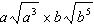Name:    Multiplying Mixed Radicals

Multiple Choice
Identify the choice that best completes the statement or answers the question.

1.

Simplify the following to a mixed radical: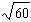a.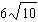c.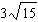b.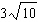d.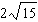2.

Simplify the following to a mixed radical: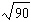a.c.b.d.3.

Simplify the following to a mixed radical: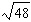a.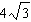c.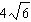b.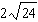d.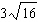4.

Simplify the following to a mixed radical: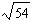a.c.b.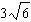d.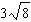5.

Multiply the following radicals: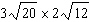a.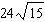c.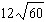b.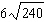d.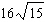6.

Multiply the following radicals: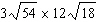a.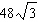c.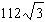b.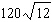d.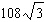7.

Multiply the following radicals: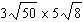a.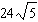c.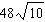b.d.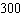Numeric Response

8.

What is the length of “x”?  Give your answer as a mixed radical in simplest form: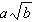--> aRb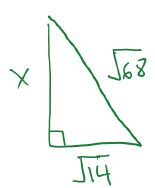9.

What is the length of “x”?  Give your answer as a mixed radical in simplest form:--> aRb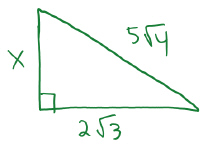10.

What is the length of “x”?  Give your answer as a mixed radical in simplest form:--> aRb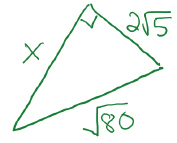11.

Find the value of side “x”: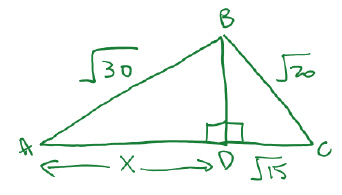12.

Find the value of side “x”: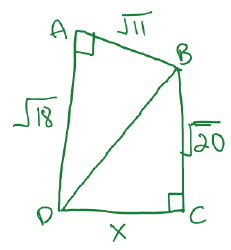Matching

 a.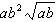e.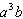b.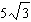f.c.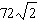g.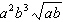d.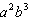h.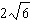13.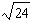14.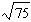15.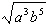16.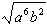17.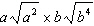18.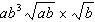19.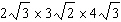20.